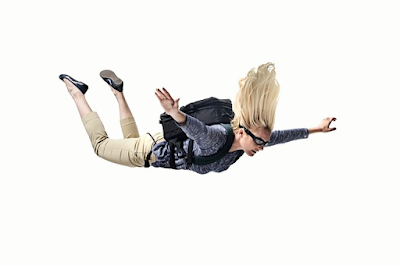Freely Falling Bodies - العلم نور

# العلم نور

{ وَقُلْ رَبِّ زِدْنِي عِلْمًا }

## Freely Falling BodiesFree falling bodies

### Free Falling Bodies

Free falling bodies refer to any objects moving up or down under the effect of its weight so, gravity is the only acceleration that acting on the moving body in that case. The earth’s gravity is a constant which equals 9.8 and take the acceleration unit of m / sec2. The gravity acceleration of any falling object always affects towards the center of the earth and is perpendicular to its surface.

In physics science, Free falling bodies motion was discussed widely and described mathematically by Newtonian equations. There are many examples of free-falling bodies in everyday life as follows:

-        Any object thrown upwards and allowed to fall freely again to the ground.
-        A ball which falls down to the earth from the second floor.

### Terminal Velocity

During the falling of the body, the speed of the body is directly proportional to the resistance of the air so, the air resistance will increase as the speed increases. When the air resistance equals the weight of the falling body, the body starts falling with a constant speed which is known as the terminal velocity.

### Equations of free-falling bodies

In these equations, the gravitational acceleration is used instead of the acceleration of normal motion. The resistance of the air is neglected as well in most cases. There are three equations for this vertical motion that are known as Newtonian equations of free-falling bodies, they are formulated as follows:
§  The first equation: v = v0 – gt
§  The second equation: y = v0t – 0.5 gt 2
§  The third equation: v 2 = v02 - 2gy
Where,
-        v0: is the initial velocity of the free-falling object (m/s).
-        v: is the final velocity of the free-falling object (m/s).
-        y: is the altitude from which the object is falling (m).
-        t: is the time of the fall (sec.).
-        g: is the acceleration of the motion due to gravity, g = 9.8 m/sec2

### Problem Solving

In all problems of free-falling bodies, you have to follow two main steps:
1.    Extract the given data carefully then choose the equation which fit your data.
2.    Substitute in this equation by the given data to get the required variables.

Example: A body is thrown upward with an initial velocity of 40 m/s. Find a) the highest point that this body will reach and b) the time which is required to reach this altitude.
The given Data:
-        v0: 40 (m/s).
-        v: 0 (m/s).
-        g: 9.8 m/sec2
The required variables:
-        y: ??? (m).
-        t: ??? (sec.).
Solution
a)    According to the given data, the third equation will be used to get the altitude:
v 2 = v02 - 2gy
02 = 402 – 2*9.8*y
y = 81.6 m
b)    The first equation will be used to calculate the time which the body requires to reach this altitude:
v = v0 – gt
0 = 40 – 9.8*t
t = 40 / 9.8
t = 4.08 sec